# Two trains

There were 159 freight wagons on the railway station creating 2 trains. One had 15 more wagons than the other. How many wagons did each train have?

a =  87
b =  72

### Step-by-step explanation:

a+b = 159
a = 15+b

a+b = 159
a-b = 15

a = 87
b = 72

Our linear equations calculator calculates it.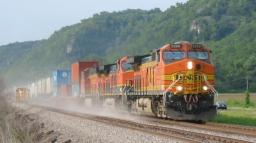Did you find an error or inaccuracy? Feel free to write us. Thank you!Tips to related online calculators
Do you have a linear equation or system of equations and looking for its solution? Or do you have a quadratic equation?

## Related math problems and questions:

• Freight train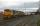The train carries 525 tons of limestone in 29 wagons. Wagons are 15 tonne and 20 tons. How many is 15 ton and how many is 20 ton wagons?
• Two trains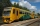From station A, the freight train traveled at a speed of 40 km/h in 9h. When he drove 15km, the fast train started from station A in the same direction at a speed of 70km/h. When will it the freight train catch up?
• The nuts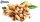There are 159 nuts in two bags, 15 more in the first than in the second, how many nuts are in the bags?
• Two trains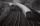There are two trains running the same distance. 1st train will travel it in 7 hours 21minutes. 2nd the train will travel 5 hours 57minutes and 34 seconds and it is 14 km/h faster than the first train. What are speeds of trains and how long is this railway
• Speed of Slovakian trainsRudolf decided to take the train from the station 'Ostratice' to 'Horné Ozorovce'. In the train timetables found train Os 5409 : km 0 Chynorany 15:17 5 Ostratice 15:23 15:23 8 Rybany 15:27 15:27 10 Dolné Naštice 15:31 15:31 14 Bánovce nad Bebravou 15:35 1
• TrainsFrom station 130 km away started passenger train and after 2.2 hours after the express train, which travels 37 km an hour more. Express train finish journey 7 minutes early. Calculate the average speed of this two trains.
• Wagons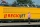We have six wagons, two white, two blue, and two red. We assemble trains from them, wagons of the same color are exactly the same, so if we change only two white wagons on a train, it's still the same train, because I don't know any different. How many di
• Siblings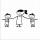Three siblings had saved up a total of 1,274 CZK. Peter had saved up to 15% more than Jirka and Hanka 10% less than Peter. How much money did they save each one of them?
• AthletesAthletes at the stadium could enter two-steps, three-steps, four-steps, five-steps, six-steps. There were more than 100 but less than 200. How many athletes were there?
• Two trains meetFrom A started at 7:15 express train at speed 85 km/h to B. From B started passenger train at 8:30 in the direction to A and at speed 55 km/h. The distance A and B are 386 1/4 km. At what time and at what distance from B the two trains meet?
• Supermarket 2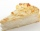A supermarket had a buko pie sale. In the morning, 2/3 of the pies were sold, and in the afternoon, 1/6 of the pies were sold. If 150 pies were left, how many pies had been sold? Show your solution.
• Two trains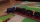Two trains departed from City A and City B against each other. They met after some time. The first train then took 9 hours to reach city B, and the second train took 4 hours to reach city A. In what proportion were the train speeds?
• Trains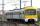On a double-track line between stations, K and M went against each other two trains. The first train passed the distance between stations for 3.5 hours, the second, which had an average speed of 12 km/h more, passed for 3.05 hours. Calculate the distance
• Saving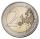The boy has saved 50 coins € 5 and € 2. He saved € 190. How many were € 5, and how many € 2?
• Dance ensembles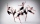4 dance ensembles were dancing at the festival. None had less than 10 and more than 20 members. All dancers from some of the two ensembles were represented in each dance. First, 31 participants were on the stage, then 32, 34, 35, 37, and 38. How many danc
• Farm2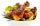The farm bred turkeys, geese, hens and chickens. Everything is 400. Turkeys and geese a hen are 150. No hen has more than one chicken, but some hens has no chick. Half of them is also a quarter of the chickens. When the turkey subtracting 15, we get 75. H
• Train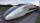A passenger train traveled for 2 hours 74 km. 3.1 hours after its departure started fast train and caught it on 186 km. How many km/h is different its average speeds?